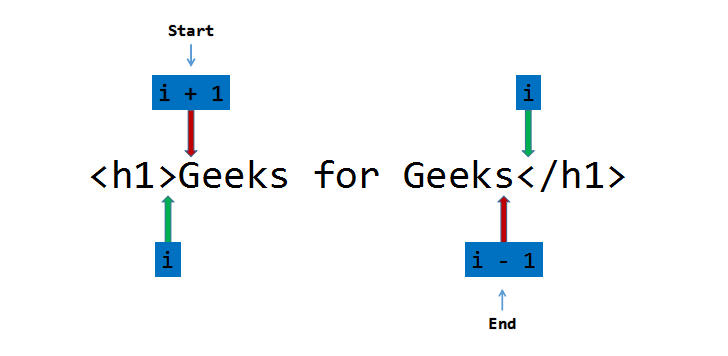GFG App
Open AppBrowser
Continue

HTML Parser is a program/software by which useful statements can be extracted, leaving html tags (like <h1>, <span>, <p> etc) behind.

Examples:

Input: <h1>Geeks for Geeks</h1>
Output: Geeks for Geeks
Explanation- <h1> and </h1> are opening and closing heading tags, so they got parsed leaving “Geeks for Geeks” as the output.

Input: <p>    Geeks for Geeks</p>
Output: Geeks for Geeks
Explanation- <p> and </p> are opening and closing paragraph tags, so they get parsed and the parser ignores space character, leaving “Geeks for Geeks” as the output.

Approach: Let the input string be S of size N. Follow the steps below to solve the problem:

• Declare two variables, start and end to point to the starting and ending point of the statement.
• Traverse the string, S uses the variable i and if S[i] is equal to ‘>’, update the start variable to i+1 and break out of the loop.
• Remove the blank spaces from the start by running a loop while S[start] is equal to ‘ ‘, and increment the start variable by 1 in each iteration.
• Again, traverse the string, S from start using the variable i and if S[i] is equal to ‘<‘, update the end to i-1 and break out of the loop.
• Run a loop and print the characters of the string S in the range [start, end].Below is the implementation of the above approach in C language:

 `// C program for the above approach ` ` `  `#include ` `#include ` `#include ` ` `  `// Function to parse the HTML code ` `void` `parser(``char``* S) ` `{ ` `    ``// Store the length of the ` `    ``// input string ` `    ``int` `n = ``strlen``(S); ` `    ``int` `start = 0, end = 0; ` `    ``int` `i, j; ` ` `  `    ``// Traverse the string ` `    ``for` `(i = 0; i < n; i++) { ` `        ``// If S[i] is '>', update ` `        ``// start to i+1 and break ` `        ``if` `(S[i] == ``'>'``) { ` `            ``start = i + 1; ` `            ``break``; ` `        ``} ` `    ``} ` ` `  `    ``// Remove the blank spaces ` `    ``while` `(S[start] == ``' '``) { ` `        ``start++; ` `    ``} ` ` `  `    ``// Traverse the string ` `    ``for` `(i = start; i < n; i++) { ` `        ``// If S[i] is '<', update ` `        ``// end to i-1 and break ` `        ``if` `(S[i] == ``'<'``) { ` `            ``end = i - 1; ` `            ``break``; ` `        ``} ` `    ``} ` ` `  `    ``// Print the characters in the ` `    ``// range [start, end] ` `    ``for` `(j = start; j <= end; j++) { ` `        ``printf``(``"%c"``, S[j]); ` `    ``} ` ` `  `    ``printf``(``"\n"``); ` `} ` ` `  `// Driver Code ` `int` `main() ` `{ ` `    ``// Given Input ` `    ``char` `input1[] = ``"

This is a statement

"``; ` `    ``char` `input2[] = ``"

This is a statement with some spaces

"``; ` `    ``char` `input3[] = ``"

This is a statement with some @ #\$ ., / special characters

"``; ` ` `  `    ``printf``(``"Parsed Statements:\n"``); ` ` `  `    ``// Function Call ` `    ``parser(input1); ` `    ``parser(input2); ` `    ``parser(input3); ` ` `  `    ``return` `0; ` `}`

Output:

```Parsed Statements:
This is a statement
This is a statement with some spaces
This is a statement with some @ #\$ ., / special characters
```

Below is the implementation of the above approach in C++ language:

 `// C++ program for the ` `// above approach ` `#include ` `using` `namespace` `std; ` ` `  `// Function to parse the ` `// HTML code ` `void` `parser(``char``* S) ` `{ ` `    ``// Store the length of the ` `    ``// input string ` `    ``int` `n = ``strlen``(S); ` `    ``int` `start = 0, end = 0; ` ` `  `    ``// Traverse the string ` `    ``for` `(``int` `i = 0; i < n; i++) { ` `        ``// If S[i] is '>', update ` `        ``// start to i+1 and break ` `        ``if` `(S[i] == ``'>'``) { ` `            ``start = i + 1; ` `            ``break``; ` `        ``} ` `    ``} ` ` `  `    ``// Remove the blank space ` `    ``while` `(S[start] == ``' '``) { ` `        ``start++; ` `    ``} ` ` `  `    ``// Traverse the string ` `    ``for` `(``int` `i = start; i < n; i++) { ` `        ``// If S[i] is '<', update ` `        ``// end to i-1 and break ` `        ``if` `(S[i] == ``'<'``) { ` `            ``end = i - 1; ` `            ``break``; ` `        ``} ` `    ``} ` ` `  `    ``// Print the characters in the ` `    ``// range [start, end] ` `    ``for` `(``int` `j = start; j <= end; j++) { ` `        ``cout << S[j]; ` `    ``} ` ` `  `    ``cout << endl; ` `} ` ` `  `// Driver Code ` `int` `main() ` `{ ` `    ``// Given Input ` `    ``char` `input1[] = ``"

This is a statement

"``; ` `    ``char` `input2[] = ``"

This is a statement with  some spaces

"``; ` `    ``char` `input3[] = ``"

This is a statement with some @ #\$ ., / special characters

"``; ` ` `  `    ``cout << ``"Parsed Statements:\n"``; ` ` `  `    ``// Function Call ` `    ``parser(input1); ` `    ``parser(input2); ` `    ``parser(input3); ` `    ``return` `0; ` `}`

Output:

```Parsed Statements:
This is a statement
This is a statement with  some spaces
This is a statement with some @ #\$ ., / special characters
```

Time Complexity: O(N)
Auxiliary Space: O(1)

Note: This program parses only one statement at a time.

My Personal Notes arrow_drop_up